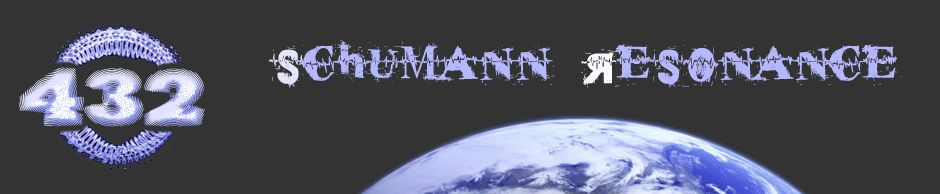# Blog » ⚠ 432Hz & The Schumann Resonance

Last Updated on

Approx. reading time: 4 minutesSeptember 27, 2013

#### 432Hz & THE SCHUMANN RESONANCE

⚠ AN IMPRACTICABLE “CIRCUMSTANTIAL” THEORY

On various sites that provide information about 432-tuning (Concert Pitch A4=432Hz or C4=256Hz + Pythagorean Temperament), the “Schumann Resonance” fundamental (at approx. 8 Hz) is often being put “on the table” as evidence: “8Hz is 5 octaves below C4=256Hz”.

HOWEVER, is it correct to state that there is a relationship between the Schumann Resonance and concert pitch A4=432Hz? Does this proclaimed “relationship” really validate the claim that using 432Hz as Concert Pitch is “better”, more “natural”, more “in sync with Earth”?

SCHUMANN RESONANCE FREQUENCY FUNDAMENTAL IS IN CONSTANT FLUX

Various sources (including Wikipedia and lunarplanner.com) make note of the resonance frequencies to be in flux, under the influence of “solar-induced perturbations to the ionosphere”. So the pitch of the resonance frequencies could even be lower or higher then the (by some 432 enthusiasts) proclaimed 8Hz.

The Schumann Resonance frequencies were first calculated by physicist Winfried Otto Schumann in 1952, he reporting the lowest in the group to be about 10 Hz. They were later measured by the National Bureau of Standards at Boulder Colorado in the 1960’s where the 7.8 Hz nominal figure was discovered along with the 5.9Hz progressing overtones.

These resonances are NOT composed of fixed or specific frequencies any more than the collective mood of human surface consciousness is fixed. Changes occurring in these frequencies are quite normal and do not indicate anything out of the ordinary. All of these frequencies fluctuate around their nominal values. For example, the fundamental Schumann frequency fluctuates between 7.0 Hz. to 8.5 Hz. These frequencies vary from geological location to location, and they can even have naturally occurring interruptions.”

In actuality, there are several frequencies between 7 and 50 Hertz that compose the Schumann Resonances. These frequencies start at 7.8 Hz and progress by approximately 5.9 Hz. (7.8, 13.7, 19.6, 25.5, 31.4, 37.3, and 43.2 Hz.).”

“There has also been rumor circulating in some esoteric circles about the 7.8 Hz. Schumann frequency increasing and that this implies a raising of the awareness or spirituality of human consciousness. In my opinion, both points are nonsense built upon misunderstandings about the fluctuating and multi-frequency nature of the Schumann Resonances and about the nature of frequencies in general. Even if it were true (which would require a significant change in the physical size of the Earth or in her surrounding atmosphere), an increase in frequency does not imply an increase in awareness — on the contrary if anything.”

Nick Anthony Fiorenza – www.lunarplanner.com

##### TOMSK (Tomsk State University Russia)

Local time in the graph below is expressed in hours of summer daylight saving time (TLDV). TLDV = UTC + 7 hours.

CLICK ON THE IMAGE BELOW (OR THE LINK ABOVE) TO SEE THE LATEST PLOTTED IMAGE!

##### VLF MONITORING STATION  (CUMIANA (TO), NW Italy, south Europe.)

Live data from The picture below shows 30 hours, and values are detected every 150s.

CLICK ON THE IMAGE BELOW (OR THE LINK ABOVE) TO SEE THE LATEST PLOTTED IMAGE!

Four traces are reported:

• Geophone trace, it shows the average and peak signals coming from the geophone sensor, in 1-20 Hz range.
• ULF noise 10 Hz, it shows the medium value between 3 and 30 Hz.
• ELF noise 100 Hz, it shows the medium value between 30 and 300 Hz.
• 2th SR frequency, it shows the frequency of second Schumann resonance (in Hz).
##### SCHUMANN FUNDAMENTAL AND MUSICAL TUNING

The idea that some 432 enthusiasts put on the table:

PYTHAGOREN @ A4=432Hz → C4=256Hz → 8Hz Fundamental.
In comparison: 12-TET @ A4=432Hz → C4=256.87Hz → 8,03Hz Fundamental.

In most sources an average of 7.8Hz is mentioned for the Schumann Fundamental. So, if we tune to C4=256Hz
(C-1=8Hz), would we then still be “in tune” with the Schumann Resonance Fundamental of 7.8Hz?

The 0.2Hz difference (in comparison with 8Hz) does not seem that much of a difference, but, when we look at 7.8Hz (C-1) and calculate C4 (the tone exactly 5 octaves above), we end up with C4=249,6Hz, a difference of 6.4Hz !!! with 256Hz. That is a rather large difference when it comes to tuning.

Other online sources do mention other frequencies when it comes to the fundamental: 7.83Hz or 7.86Hz. With only a decimal difference of .03Hz or .06Hz the result of the tone Frequency 5 octaves above, would generate a significant difference:

7.80Hz fundamental → C4=249.60Hz → A4=419.77Hz (12-TET) / A4=421.19Hz (Pythagorean)
7.83Hz fundamental → C4=250.56Hz → A4=421.39Hz (12-TET) / A4=422.82Hz (Pythagorean)
7.86Hz fundamental → C4=251.51Hz → A4=422.99Hz (12-TET) / A 4=424.42Hz (Pythagorean)
8.00Hz fundamental → C4=256.00Hz → A4=430.54Hz (12-TET) / A4=431.99Hz (Pythagorean)
8.03Hz fundamental → C4=256.89Hz → A4=432Hz (12-TET) / A4=433.5Hz (Pythagorean)

#### CONCLUSION …

Using the Schumann Resonance as “solid evidence” to “prove” the validity of A4=432Hz as concert pitch is rather “circumstantial” (some would say “pseudo-scientific”).

HOWEVER, since resonance is in flux, then it is reasonable to say the fundamental “can be”, “could become”, and “might have been” at exactly 8Hz at various (short) moments in time. So, from “moment to moment” using A4=432Hz might make your music “in sync” with the Schumann Resonance fundamental frequency, with “Earth’s vibratory nature” … and “out of sync” at other moments too.

And so does 440Hz at moments … when in flux the pitch rises …

12-TET: A4=440Hz → C4=261.63Hz → 8.18Hz fundamental.
PYTHAGOREAN: A4=440Hz → C4=260.74Hz → 8.15Hz fundamental.

As you can see, 8.18Hz and 8.15Hz in the examples above is well within the flux range of the Schumann Resonance Fundamental. You could say that 440Hz is equally valid as Concert Pitch as 432Hz if the Schumann Resonance Fundamental is “leading proof”. 😉

REFERENCES: